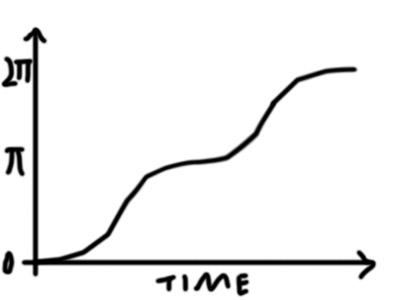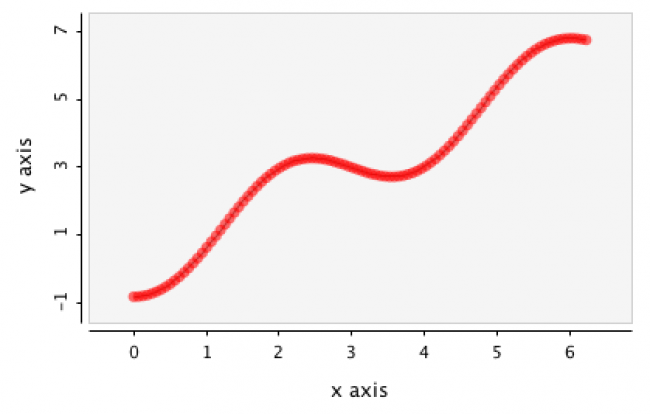#### Howdy, Stranger!

We are about to switch to a new forum software. Until then we have removed the registration on this forum.

# Continuous Eased Flip/Rotation

edited January 2018

I want to move a shape around in 3D continuously, but I want it to spend most of the time close to perpendicular to the camera.

I'm looking for a motion something like this:I have the following code, which does what I want it do for now:

``````int flipTime;
float rotation;

void setup() {
size(400, 400, P3D);
rotation = 0.0;
flipTime = 100;
}

void draw() {

background(128);
translate(200, 200, 20);

if ( (frameCount % flipTime) < (flipTime/2) ) {
rotation = HALF_PI * ( 1+ sin( ( (frameCount % (flipTime/2)) * PI / (flipTime/2) ) -HALF_PI) );
} else {
rotation = PI + (HALF_PI * ( 1+ sin( ( (frameCount % (flipTime/2)) * PI / (flipTime/2) ) -HALF_PI) ));
}

rotateY(rotation);

fill(255);
box(200, 200, 20);
fill(0);
translate(0, 0, -20);
box(200, 200, 20);
}
``````

However, the if/else structure seems like it's going to make things complicated further down the line. I'd like to stack many such rotations and translations on different axes and apply them to the same object, all out of phase with each other, and be able to tweak the variables, maybe using sin(x)^3, sin(x)^5 etc. for more eased motion.

Is there a more elegant way to do this?

Also, is there a page somewhere that gives examples on how to manipulate sine functions for these kinds of purposes? Something like the Trigonometry Primer, but part 2?

Tagged:

• A demo in 2D below.

I'd like to stack many such rotations and translations on different axes and apply them to the same object, all out of phase with each other

Step 1: Different rotations on different axis
Step 2: Those rotations out of phase wrt each other

For step 1:
rotateY(rotation);
rotateX(rotation);
rotateZ(rotation);

For step 2:
rotateY(rotation+phaseY);
rotateX(rotation+phaseX);
rotateZ(rotation+phaseZ);

where the phases are defined in setup().

Kf

``````//REFERENCES: https://forum.processing.org/two/discussion/25941/continuous-eased-flip-rotation#latest
//REFERENCES:
//REFERENCES:

//INSTRUCTIONS:
//         *--  Press the SPC key to toggle between manual or auto mode
//         *--  In automode, the rect rotates manually based on demo curve
//         *--  In manual mode, rect rotate based on position of mouse accross
//         *--  sketch width (Tip: Follow the curve with the mouse)

//===========================================================================
// IMPORTS:

//===========================================================================
// FINAL FIELDS:

//===========================================================================
// GLOBAL VARIABLES:

int N=100;    //Number of points to plot
float amp=50; //Amplitude of sine wave

float ctr=0;  //Counter for rect rotation: Manual or auto rotation

boolean manualFlag=false;

//===========================================================================
// PROCESSING DEFAULT FUNCTIONS:

void settings() {
size(600, 600);
}

void setup() {

textAlign(CENTER, CENTER);
rectMode(CENTER);

fill(255);
strokeWeight(2);
noStroke();
//noLoop();
}

void draw() {
background(0);
fill(255);
for (int i=0; i<N; i++) {
float x=map(i, 0, N, 0, width);
float y=map(i, 0, N, 0, height);
;
y=height-y+amp*sin(map(i, 0, N, 0, 2*TWO_PI));
}

float y=map(ctr, 0, N, 0, height);
y=height-y+amp*sin(map(ctr, 0, N, 0, 2*TWO_PI));  //Linear plus sin wave
float yp=map(y, 0, height, 0, TWO_PI);
translate(width/2, height/2);
rotate(yp);
fill(200, 25, 0);
rect(0, 0, 70, 50);

if (manualFlag==true)
ctr=map(mouseX, 0, width, 0, N-1);
else
ctr=(ctr+1)%N;
}

void keyReleased() {
if (key==' ') {
manualFlag=!manualFlag;

//if(manualFlag)
ctr=0;
}
}

void mouseReleased() {
}
``````
• edited January 2018 Answer ✓

@PJMcPrettypants -- instead of components, let's try an additive approach -- superimposing waves. Rotate your graph by 45 degrees and look at it. It looks like a flat sine wave, right? https://en.wikipedia.org/wiki/Sine_wave

``````y(t) = A*sin(2pi*f*t+p)
``````

Now we just need to put it on a slope. Here is a slope:

``````y(t) = t
``````

...so let's have the waves go up and down from that slope as the baseline:

``````y(t) = t + A*sin(TWO_PI*f*t+p)
``````

But what if the slope is too steep -- you wanted it to take longer!

``````y(t) = slope*t + A*sin(TWO_PI*f*t+p)
``````

Ok, but that curve is way too loud! Let's make the sign wave flatter by dropping the amplitude, just like we would if it wasn't on a curve:

``````y(t) = 0.5*sin(TWO_PI*f*t+p)
``````

...but wait -- the frequency needs to be higher, and the phase isn't aligning the curves correctly! No problem -- just manipulate f and p in your sine component to change alignment....

• To play around with settings on a labeled graph, drop this into the setup() of the grafica DefaultPlot sketch and start tweaking settings:

``````      float A=1.0;
float f=HALF_PI+QUARTER_PI/4;
float p=-HALF_PI-QUARTER_PI/2-QUARTER_PI/4;
float t=0;
for (int i = 0; i < nPoints; i++) {
t = i * (TWO_PI/nPoints);
points.add(t, t + (A * sin(t * f + p)));
}
``````

Note that this particular approach (sin + x=y) may not specifically work out for what you have in mind, but it gives you a general idea of how to add things together to get a motion profile.• Thanks to both of you.

One thing that tripped me up with the linear plus sine function was trying to change the variables but keep the flat parts of the curve locked in on 0, pi, 2pi, etc.

What I didn't realise was that the frequency and slope have to stay in proportion to one another to hit those spots.

So in

`y(t) = slope*t + A*sin(f*t+p)`

frequency has to be 2*slope.

Also phase has to be pi, and if amplitude goes over 0.5 the rotation will change direction rather than just slow down.

So it could be written as

`y(t) = slope*t + 0.5*sin(slope*2*t+PI)`

I've changed my original sketch so that the rotation time is controlled by mouseX:

``````int flipTime; //time in frames for complete rotation
float amplitude;
float slope;

void setup() {
size(400, 400, P3D);
amplitude = 0.5;
}

void draw() {

background(128);
translate(200, 200, 20);

flipTime = mouseX;
slope = TWO_PI/flipTime;

rotateY((slope * frameCount) + (amplitude * sin((frameCount * slope *2) + PI) ));

fill(255);
box(200, 200, 20);
fill(0);
translate(0, 0, -20);
box(200, 200, 20);

}
``````
• @PJMcPrettypants -- Thanks for sharing your solution and demo! Very nice timed flipping effect.